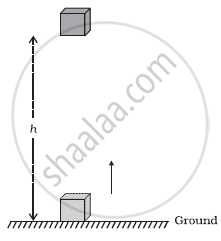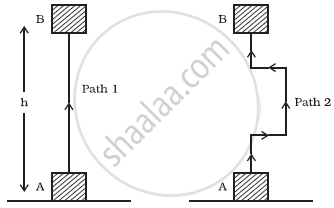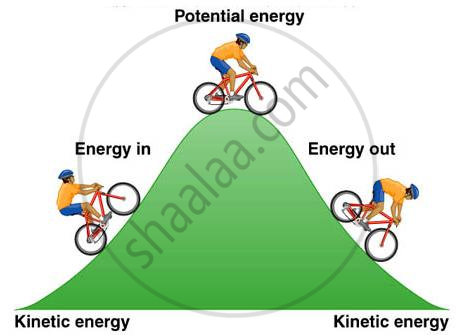# Types of Mechanical Energy: Potential Energy

### POTENTIAL ENERGY OF AN OBJECT AT A HEIGHT:

An object increases its energy when raised through a height. This is because work is done on it against gravity while it is being raised. The energy present in such an object is the gravitational potential energy. The gravitational potential energy of an object at a point above the ground is defined as the work done in raising it from the ground to that point against gravity. It is easy to arrive at an expression for the gravitational potential energy of an object at a height.

Consider an object of mass, m. Let it be raised through a height, h from the ground. A force is required to do this. The minimum force required to raise the object is equal to the weight of the object, mg. The object gains energy equal to the work done on it. Let the work done on the object against gravity be W. That is, work done, W = force × displacement = mg × h = mgh Since work done on the object is equal to mgh, an energy equal to mgh units is gained by the object. This is the potential energy (EP) of the object. Ep = mghIt is useful to note that the work done by gravity depends on the difference in vertical heights of the initial and final positions of the object and not on the path along which the object is moved.

The block is raised from position A to B by taking two different paths. Let the height AB = h. In both the situations the work done on the object is mgh.

#### notes

Potential Energy:

Every object possesses some energy called Potential Energy. An object when gains energy may store it in itself as potential energy.We know that when an object rises above the ground some work is done against gravity. Since work is done on the object, the object would gain some energy. The energy that the object gains at a height is called Gravitational Potential Energy. It is defined as the amount of work done required in raising an object above the ground up to a certain point against the gravity.

W = F x d = F x h (height)

And F = m x g (because the force is applied against gravity)

So, W = m x g x h

Hence potential energy of object A, Ep = m x g x h

Elastic Potential energy: Same work has to be done to change the shape of a body. This work gets stored in the deformed body in the form of elastic potential energy. Elastic potential energy is never negative whether due to extension or to compression.

#### description

• Potential Energy
• Expression for the potential Energy
• Some examples of potential energy Gravitational potential energy
If you would like to contribute notes or other learning material, please submit them using the button below.

### Shaalaa.com

Potential Energy [00:07:03]
S
0%